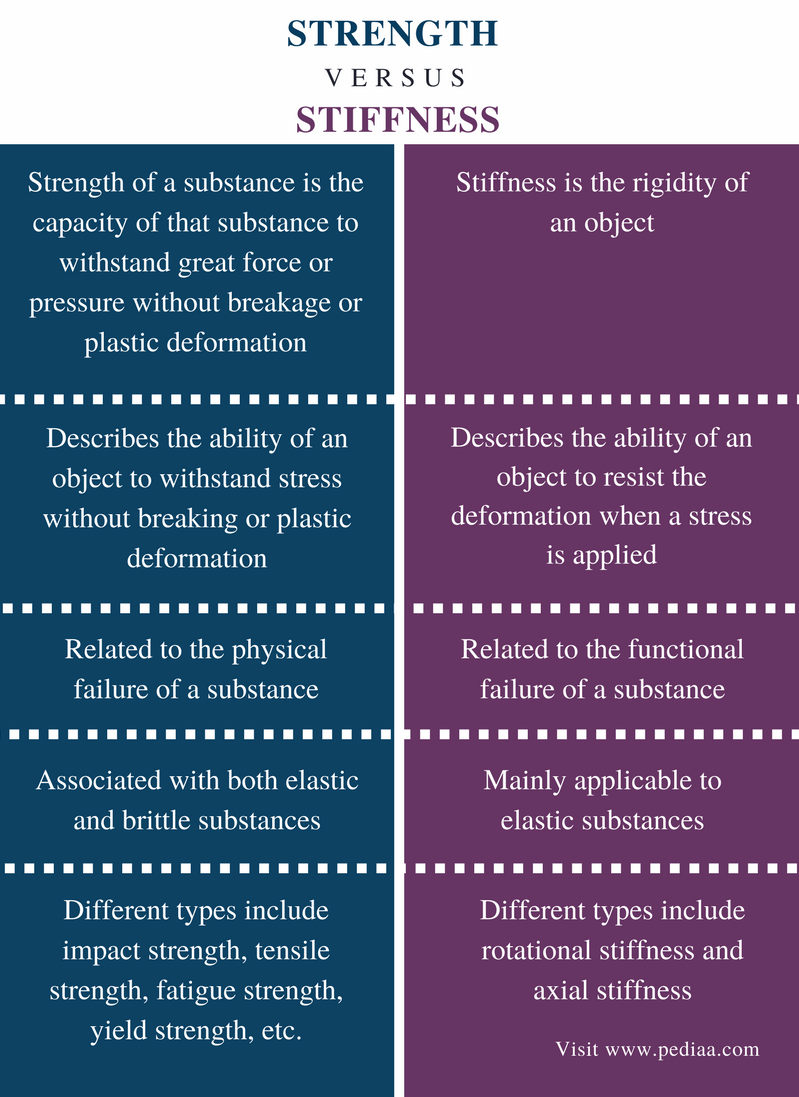# Difference Between Strength and Stiffness

## Main Difference- Strength vs Stiffness

Strength and stiffness are two physical properties of materials. Both terms describe how strong the material is, but have different definitions. Strength is the capacity of an object or substance to withstand great force or pressure. Stiffness is the rigidity of an object (the extent to which it resists deformation in response to an applied force). Therefore, there is a difference between strength and stiffness, though they are used interchangeably. The main difference between strength and stiffness is that strength is the ability of an object to withstand stress without breaking whereas stiffness is the ability of an object to resist deformation when a stress is applied.

### Key Areas Covered

1. What is Strength
– Definition, Different Types, Deformation
2. What is Stiffness
– Definition, Different Types, Formula
3. What is the Difference Between Strength and Stiffness
– Comparison of Key Differences

Key Terms: Fatigue Strength, Impact Strength, Stiffness, Strength, Tensile Strength, Yield Strength## What is Strength

Strength of a substance is the capacity of that substance to withstand great force or pressure without breakage or plastic deformation. Plastic deformation is the permanent deformation or change in the shape of a solid body without fracture under the action of a sustained force. Therefore, the term strength describes the ability of a substance to support a force without any physical failure.

There are several different terms used to describe strength. All these terms explain some specific physical properties of matter. Some of these terms are given below.

• Impact strength – the ability of a substance to withstand suddenly applied force.
• Tensile strength – the maximum stress that a substance can withstand without being stretched or breakage.
• Yield strength – the minimum stress required to make a permanent deformation in a substance
• Fatigue strength – the highest stress a material can withstand for a given number of cycles without breaking. This is also known as endurance strength.

There are different types such as ductile substances, brittle substances, elastic substances, etc. The strength of these substances varies depending on the type of substance. For example, the strength of a brittle substance is the capacity of that substance to withstand an applied stress without breakage whereas the strength of a ductile substance is the capacity of that substance to withstand an applied stress without any plastic deformation.Figure 1: Typical Stress-Strain Curve for a Ductile Material

The above curve shows the stress-strain curve for a ductile material. A ductile material is a substance that can be drawn into thin wires without breakage. The yield strength gives the minimum strength required to permanently deform the material into a wire. Ultimate strength is the maximum strength the material can withstand while deforming.

## What is Stiffness

Stiffness is the rigidity of an object. Rigidity refers to the ability to resist deformation. It the resistance to functional failure. Stiffness is the opposite of flexibility. More flexible a substance is, less stiff it is. This term is mainly used for elastic substances since brittle substances do not undergo any deformation.

Stiffness can be expressed as follows:

K  =  F / δ

• K is the stiffness
• F is the applied force
•  δ is the deformationFigure 2: Stiffness of a Substance that Can be Bent

Stiffness is given by the unit N/m. This is because the unit for applied force is N (Newton) and the deformation is given by m (meters). This deformation is often a change in length. There are several types of stiffness.

• Rotational stiffness – this is given by the applied moment divided by the rotation
• Axial stiffness – this is given for an object in tension or compression.

## Difference Between Strength and Stiffness

### Definition

Strength: Strength of a substance is the capacity of that substance to withstand great force or pressure without breakage or plastic deformation.

Stiffness: Stiffness is the rigidity of an object.

### Ability

Strength: Strength describes the ability of an object to withstand stress without breaking or plastic deformation.

Stiffness: Stiffness describes the ability of an object to resist the deformation when a stress is applied.

### Failure

Strength: Strength is related to the physical failure of a substance.

Stiffness: Stiffness is related to the functional failure of a substance.

### Substances

Strength: Strength is associated with both elastic and brittle substances.

Stiffness: Stiffness is mainly applicable to elastic substances.

### Different Types

Strength: Different types of strengths include impact strength, tensile strength, fatigue strength, yield strength, etc.

Stiffness: Different types of stiffness include rotational stiffness and axial stiffness.

### Conclusion

Strength and stiffness are two physical properties of substances. The main difference between strength and stiffness is that strength is the ability of an object to withstand a stress without breaking whereas stiffness is the ability of an object to resist the deformation when a stress is applied.

##### Reference:

1. “Strength of materials.” Wikipedia, Wikimedia Foundation, 13 Jan. 2018, Available here.
2. “Physical Properties Testing: Tensile Strength, Flexural Strength, Impact.” ELTEK International Laboratories, Available here.
3. Stiffness. Engineering Tool Box, Available here.

##### Image Courtesy:

1. “Beam Stiffness” By Ootsuka02 – Own work (CC BY-SA 3.0) via Commons Wikimedia
2. “Stress Strain Ductile Material” By Breakdown – Own work (CC BY-SA 3.0) via Commons Wikimedia